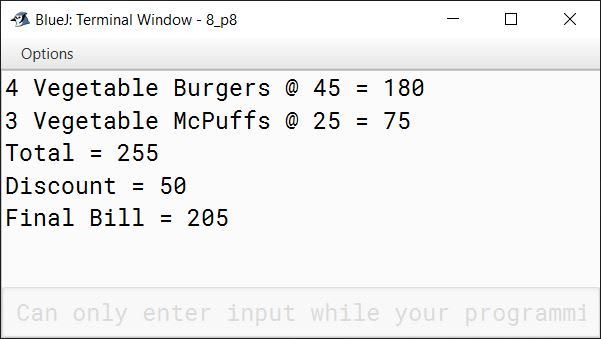# Introduction to Java & BlueJ

## Fill in the blanks

#### Question 1

The Java programming language is an Object Oriented Programming language.

#### Question 2

Encapsulation is the technique of binding both data and functions together.

#### Question 3

A Class is a blueprint that defines data and functions common to all objects of a certain kind.

#### Question 4

The smallest meaningful element of a Java program is called a Token.

#### Question 5

Initialization is a process of assigning some initial value to a variable.

## State True or False

#### Question 1

Polymorphism allows a function to behave differently for different objects.
True

#### Question 2

Constants mean the fixed values that do not change during the execution of a program.
True

#### Question 3

The main() function indicates that the execution of the Java program will begin from this point.
True

#### Question 4

Every Java statement must end with a colon.
False

#### Question 5

Inheritance is a feature using which an object in one class acquires the properties of another class.
True

#### Question 6

While comparing two variables, their data types should not be the same.
False

## Application Based Questions

#### Question 1

Ritika has written a program in Java. After the successful compilation of her program, which option should she select to run it?

To run her program, Ritika needs to right-click on the class icon and select void main(String[] args) option from the pop-up menu.

#### Question 2

Kareena is writing a program in Java to calculate the average of three subjects. Which operators will she use for the same?

Kareena will use the addition operator (+) to first calculate the total marks obtained in all three subjects. After that, she will use the division operator (/) to divide the total marks by 3 to get the average.

## Multiple Choice Questions

#### Question 1

A .......... is a named location in the memory, which stores data temporarily.

1. Identifier
2. Keyword
3. Variable ✓

#### Question 2

What does OOP mean?

1. Object Oriented Programming ✓
2. Object Oriented Procedure
3. Object Origin Program

#### Question 3

Which among the following feature is used to manage the complexity of the system?

1. Abstraction ✓
2. Polymorphism
3. Encapsulation

#### Question 4

A Java program is compiled into an intermediate language called ..........

1. Source Code
2. Bytecode ✓
3. None of these

#### Question 5

The .......... works on a single variable or constant.

1. Unary Operator ✓
2. Arithmetic Operator
3. Relational Operator

#### Question 6

Which symbol is used to combine two or more items in a single line?

1. ?
2. + ✓
3. !

#### Question 1

Define objects. Give a real life example to explain objects along with their attributes and behaviour.

An object represents data (attributes) and its related methods (behaviour) as a single unit. Take the example of a car. When we describe a car as an object, there are a number of physical factors which contribute to define its state such as its brand name, colour, speed, size, number of seats, etc. In addition, the car has several functional definitions. It transports people to different locations by controlling its speed and direction, an accelerator to increase or decrease its speed and brakes to stop it. Thus we can say that car is an object that combines its attributes and behaviour as a single unit.

#### Question 2

What is class?

A class is a blueprint to create objects. It defines data and functions common to all objects of a certain kind.

#### Question 3

What do you understand by the term Polymorphism?

The word Polymorphism means "many forms". Polymorphism helps the programmer to use the same function name for more than one purpose.

#### Question 4

What are operators? Why do we need them?

Operators are special symbols that are used to perform calculations. We need operators to form expressions by applying them to the variables and constants.

#### Question 5

What do you understand by the term Keyword?

Keywords are reserved words that have a special meaning for the Java compiler. Java compiler reserves these words for its own use so Keywords cannot be used as identifiers. For example, void, public, class, int, etc.

#### Question 6

What are variables? How are they different from Constants?

A variable is a named location in the memory which stores data temporarily. A variable has a unique name, a type and a size that is used to identify it in a program.

Difference between variables and constants is that the value of variables can change during the execution of the program whereas value of constants are fixed and does not change during program execution.

## Lab Session

#### Question 1

Write a program to print, "Welcome to the World of Computers".

```public class KboatWelcome
{
public static void main(String args[]) {
System.out.println("Welcome to the World of Computers");
}
}```
##### Output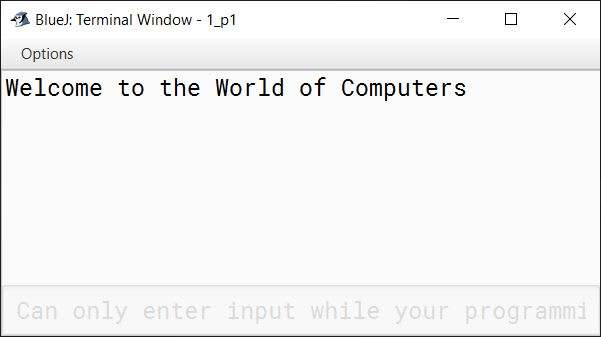#### Question 2

Write a program to print your Name, Class, Roll_No, Marks and Age. Name this file, `MyProfile'.

```public class MyProfile
{
public static void main(String args[]) {
System.out.println("Name: Sneha Nayak");
System.out.println("Class: 8C");
System.out.println("Roll No: 24");
System.out.println("Marks: 87");
System.out.println("Age: 15");
}
}```
##### Output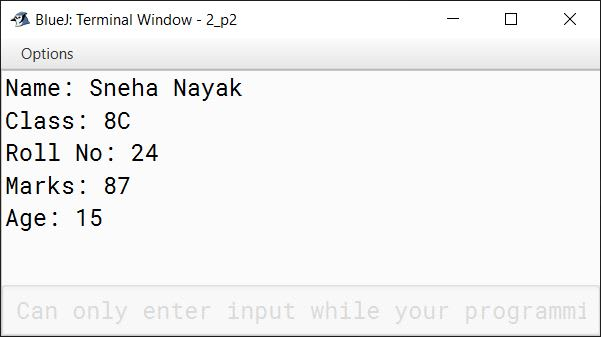#### Question 3

Write a program to print your School name four times in separate lines.

```public class KboatSchoolName
{
public static void main(String args[]) {
System.out.println("St. Francis' College");
System.out.println("St. Francis' College");
System.out.println("St. Francis' College");
System.out.println("St. Francis' College");
}
}```
##### Output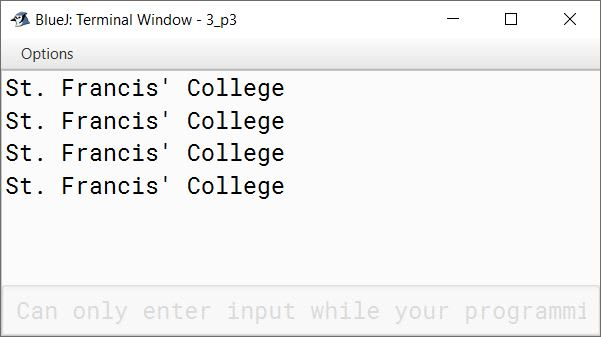#### Question 4

Write a program to find the sum and average of three numbers.

```public class KboatSumAvg
{
public static void computeSumAvg(int a, int b, int c) {
System.out.println("The three numbers are "
+ a + ", " + b + ", " + c );
int sum = a + b + c;
double avg = sum / 3.0;
System.out.println("Sum = " + sum);
System.out.println("Average = " + avg);
}
}```
##### Output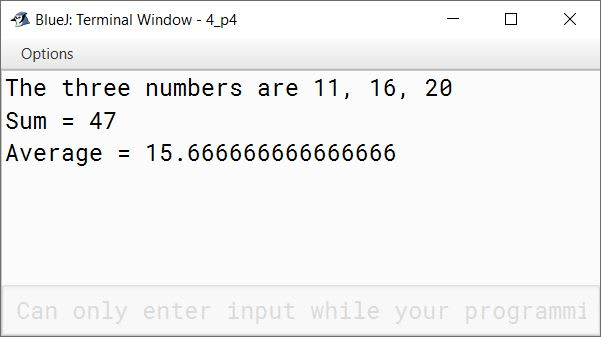#### Question 5

Write a program to interchange the value of two numbers without using the third variable.

```public class KboatSwap
{
public static void swapNumbers(int a, int b) {
System.out.println("The numbers are:");
System.out.println("a=" + a + " b=" + b);
a = a + b;
b = a - b;
a = a - b;
System.out.println("The numbers after interchange:");
System.out.println("a=" + a + " b=" + b);
}
}```
##### Output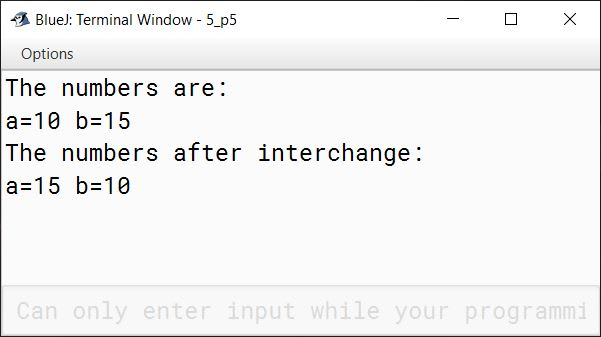#### Question 6

Write a program to calculate the compound interest.

```public class KboatInterest
{
public static void computeInterest(double p, double r, int t) {
double amt = p * Math.pow(1 + (r / 100 ), t);
double interest = amt - p;
System.out.println("Compound Interest = " + interest);
}
}```
##### Output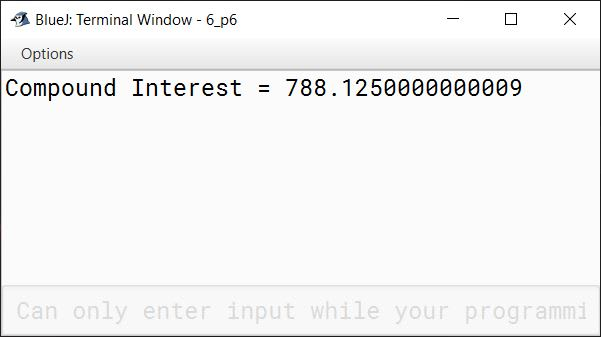#### Question 7

Write a program to calculate the tax for a taxable income of Rs. 4,10,000, if the tax rate is fixed at 3.2%.

```public class KboatTax
{
public static void main(String args[]) {
int income = 410000;
double rate = 3.2;
double tax = income * rate / 100;
System.out.println("Tax = " + tax);
}
}```
##### Output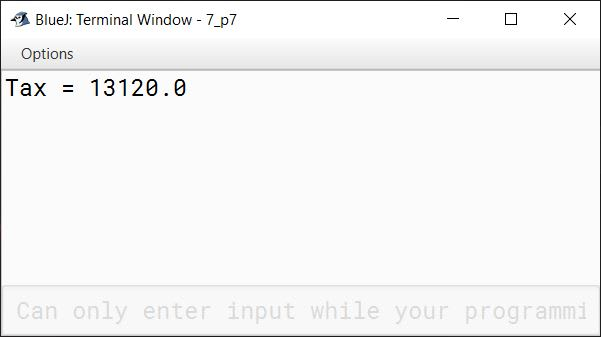#### Question 8

Create a program that will generate a bill at McDonald's for four vegetable burgers (@ Rs 45 per vegetable Burger) and three vegetable McPuffs (@ Rs 25 per vegetable McPuff). There is a special Independence Day discount of Rs 50 on the final bill amount.

```public class KboatMcDBill
{
public static void main(String args[]) {
int vegBurgerCost = 4 * 45;
int vegMcPuffCost = 3 * 25;
int total = vegBurgerCost + vegMcPuffCost;
int amt = total - 50;
System.out.println("4 Vegetable Burgers @ 45 = " + vegBurgerCost);
System.out.println("3 Vegetable McPuffs @ 25 = " + vegMcPuffCost);
System.out.println("Total = " + total);
System.out.println("Discount = 50");
System.out.println("Final Bill = " + amt);
}
}```
##### Output